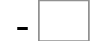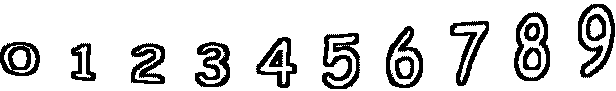1= 1   3= 09= 1   7= 3   0= 0   5= 3 8= 7   8= 7   8= 5   1= 1